Triangle Similarity
Triangle Proportionality Theorem
Dilations
Using Similar Triangles
100

Are the two triangles similar? If so, state the theorem that justifies your answer.AA, Triangle Similarity

100

Find the missing length.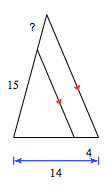6

100

What are the coordinates of the point K(4, -3) after a dilation with a scale factor of 5?

K'(20, -15)

100

3 feet

200

Are the two triangles similar? If so, state the theorem that justifies your answer.SSS, Triangle Similarity

200

Find the missing length.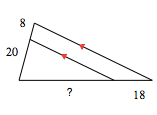45

200

What are the coordinates of the point B(8, -20) after a dilation with a scale factor of k=1/8?

B'(1, -2.5)

200

A 40-foot flagpole casts a 25-foot shadow. Find the shadow cast by a nearby building 200 feet tall.

125 feet

300

Are the two triangles similar? If so, state the theorem that justifies your answer.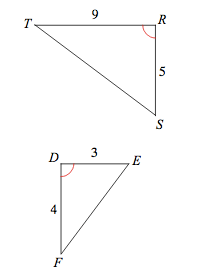Not Similar

300

Find the missing length.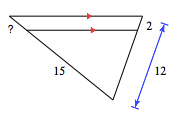3

300

What is the scale factor k used if L(-2, 6) becomes L'(-6, 18)?

k=3

300

Roger wants to find the height of the flagpole. Roger is 5 feet 6 inches tall and casts a shadow about 3 feet long. The flagpole casts a shadow about 11 feet long. Use this information to help Roger find the height of the flagpole, in inches.

242 inches (20 ft 2 ins)

400

Are the two triangles similar? If so, state the theorem that justifies your answer.SAS, Triangle Similarity

400

Solve for x.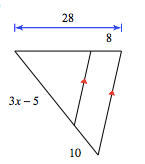x = 10

400

What is the scale factor k used if S(-18, 4) becomes S'(-9, 2)?

k=1/2

400

Ryan and Jack are standing opposite each other. Ryan places a mirror on the ground between them so from where he is standing, he can to the top of Jack's head the in the reflection. If Ryan is 6 feet tall, Jack is 6 feet 6 inches tall, and Ryan is 5 1/2 feet from the mirror, how far is Jack from the mirror, in inches?

71.5 inches

500

Are the two triangles similar? If so, state the theorem that justifies your answer.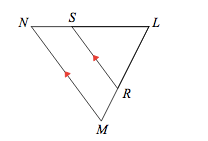AA, Triangle Similarity

500

Find the missing length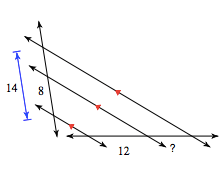9

500

An 8x12 photograph was enlarged to 12x18.  What was the scale factor k used to produce the larger photograph?

k=1.5 or k=3/2

500

Elizabeth’s eyes are 150 cm from the floor. She places a mirror on the floor 18 m from the base of a climbing wall. She walks backward 120 cm, until she sees the top of the wall in the mirror. What is the height of the climbing wall, in cm?

2250 cm

Click to zoom